# HBSE 9th Class Science Important Questions Chapter 4 Structure of the Atom

Haryana State Board HBSE 9th Class Science Important Questions Chapter 4 Structure of the Atom Important Questions and Answers.

## Haryana Board 9th Class Science Important Questions Chapter 4 Structure of the Atom

Question 1.
How are materials formed?
Materials are formed with the combination of atoms and molecules.

Question 2.
What is known as Atom?
Atom is the microscopic indivisible particles that have an independent existence.

Question 3.
During which century did Dalton agree an atom to be indivisible?
During 19th century, Dalton agreed an atom to be indivisible.

Question 4.
Which are the basic particles of an atom ?
The basic particles of an atom are electrons, protons and neutrons.

Question 5.
Define isobars with examples.
Atoms of different elements which have same mass number but different atomic numbers are known as isobars. For example, calcium (20Ca40) and argon (18Ar40) are isobars.

Question 6.
What is meant by electron ?
In an atom, the negatively charged particle whose mass is $$\frac {1}{2000}$$ part mass of a proton that revolves around the nucleus is called an electron.

Question 7.
Which scientist gets the honor of discovering electron?
J.J. Thomson.

Question 8.
Which charge does remain on an electron?
Negative (-ve) charge.

Question 9.
Which scientist discovered the canal Rays?
The German scientist, E. Goldstein.Question 10.
Which other name is called as the canal rays ?
Canal rays are also called as positive rays.

Question 11.
Which electron are canal rays attracted towards in the electronic field ?
Towards negative electron.

Question 12.
How are electron and proton represented ?
Electron is represented by e~ and proton is represented by p+.

Question 13.
What is the mass and charge of proton ?
The mass of proton is 1 unit and the charge is +1.

Question 14.
How much is the charge on an electron ?
An electron has -1 charge.

Question 15.
Why is atom electrically neutral ?
Because in an atom the number of electrons and protons mutually balance the charges.

Question 16.
Which discovery enabled J.J. Thomson to win the Nobel Prize in Physics ?
For discovery of‘electron’.

Question 17.
When was J.J. Thomson born ?
J.J. Thomson was born on 18 December, 1856.

Question 18.
What is the mass of‘alpha’ particles ?
The mass of ‘alpha’ particles is 4u.Question 19.
What are alpha particles?
Alpha particles are binary-charged helium particles.

Question 20.
Which scientist is known as the father of nuclear physics?
E. Rutherford is called as the father of nuclear physics.

Question 21.
When was E. Rutherford born?
E. Rutherford was born on 30 August 1871.

Question 22.
According to Rutherford, where does the entire mass of an atom lie in ?
In the micronucleus of the atom.

Question 23.
Compare the size of the nucleus with respect to that of the size of an atom.
The size of the nucleus is 1O3 times less than that of the size of an atom.

Question 24.
Which charge does atom-nucleus have?
Positive charge.

Question 25.
Of which element’s nucleus is known as proton?
Of hydrogen nucleus.

Question 26.
What was the proposal of Neils Bohr regarding the revolving of electrons?
According to Neils Bohr electrons revolve in a certain energy orbit.

Question 27.
According to Neils Bohr model, when an electron returns from a higher level orbit to a lower level orbit, then the difference of energy gets radiated into which form?
Electromagnetic radiation or in the form of light.

Question 28.
Which particles does the nucleus of an atom have?
Protons and Neutrons.Question 29.
How many a maximum number of electrons can be accommodated in the first and second orbit of an atom?
In the first orbit, two and in the second orbit, maximum of eight electrons can be accommodated.

Question 30.
Write down the name of the lightest atom.
Hydrogen.

Question 31.
Write down the name of that atom which does not have any neutron.
Protium (11H ) which is an isotope of hydrogen.

Question 32.
Write down the number of proton and neutron present in the nucleus of helium.
There are two protons and two neutrons in the nucleus of helium.

Question 33.
When and who discovered the neutron ?

Question 34.
In the structure of atom the electrons of which orbit determine the properties of atoms.
The electrons in the last shell (valence shell).

Question 35.
In a single molecule of water, how many atoms of hydrogen and oxygen are there?
In a single molecule of water, there are two atoms of hydrogen and one atom of oxygen.

Question 36.
Which atoms are chemically active?
The atoms whose outermost shells are not complete are chemically active.

Question 37.
Which atoms are chemically neutral?
Those atoms whose outermost shells are complete are chemically neutral. For example; helium and neon.

Question 38.
Give names of two bivalent atoms.
(i) Calcium (Ca)
(ii) Berkelium (Be).Question 39.
What is the valency of chlorine?
One.

Question 40.
What will be the number of neutrons in the atom of the element whose atomic number is 19 and mass number is 39?
Neutron = mass number – atomic number = 39 – 19 = 20

Question 41.
Why is the mass of the atom M and its ion M+ same?
Because both have same number of neutrons and protons.

Question 42.
Write dow n two isotopes of uranium.
23592U and 23892U

Question 43.
Which two of the following nuclei are isotopes mutually?
90Z231, 91Z230, 88Z230, 90Z233
90Z231 and 90Z233

Question 44.
How many isotopes does carbon have ?
Carbon has two isotopes 12 C and 14 C .

Question 45.
What are the atoms called that belong to only one element, which has same atomic number but different mass number?
Isotopes.

Question 46.
What is the average mass of isotopes of chlorine?
The average mass of isotopes of chlorine used to be 35.5u.

Question 47.
Which of the followings are nuclear isotopes?
88Z226, 87Z236,88Z238,88Z238
88Z226, 88Z238,

Question 48.
Which radioactive isotope is used in the treatment of cancer ?
Isotope of cobalt of mass number 60.

Question 49.
Which fuel is used in a nuclear plant ?
In a nuclear plant, isotope of uranium (U-235) is used as fuel.

Question 50.
Which elements isotopes are used in treatment of goitre disease ?
Isotopes of iodine are used in the treatment of goitre disease.

Question 51.
Write down names of two isobaric elements.
Calcium and argon are isobaric elements.Question 1.
Why are canal rays called as positive charged rays ?
Falling of the canal rays towards negative electrons in the electric field shows that these rays are jointly made up of positively charged particles.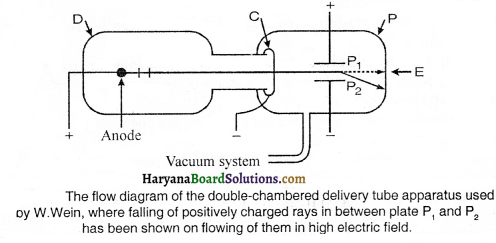Question 2.
Write down the properties of canal rays.
Following are the properties of canal rays:
(1) These rays move in a straight line.
(2) These rays are bent towards negative electrode in electric and magnetic field.
(3) They have mass many times more than the mass of electron.

Question 3.
Why are electrons called as planetary electrons in an atom ?
In an atom, electrons revolve in their respective fixed large elliptical orbits just like planets in the sky revolve around the sun in their large elliptical orbits. Hence, electrons are called as planetary electrons.

Question 4.
Enunciate similarities and dissimilarities between protons and neutrons.
Similarities between protons and neutrons:
(1) Mass of proton and neutron is approximately equal to a single atomic mass unit.
(2) Proton and neutron both exist in the nucleus of an atom.

Dissimilarities between protons and neutrons:
(1) Protons are positively charged particles, whereas neutrons are neutral particles.
(2) The mass of a neutron is somewhat more than that of the mass of a proton.

Question 5.
Write down the difference between electron and neutron.
Following is the difference between electron and neutron.

Electron:
1. Electron possesses negative (-ve) charge.
2. They revolve around in different orbits outside the nucleus.
3. Their mass is the 1/2000th part by mass of a hydrogen atom.

Neutron:
1. Neutron possesses negligible charge.
2. They remain inside the nucleus.
3. Their mass is almost the same to that of the mass of an atom of hydrogen.

Question 6.
Write down the difference between electron and proton.
Following is the difference between electron and proton :
Electron:
1. They possess unit (-ve) charge on them.
2. Their mass is l/’2000th part mass of an atom of hydrogen.
3. They revolve around in their respective orbits outside the nucleus.

Proton:
1. They possess unit (+ve) charge on them.
2. Their mass is almost the same to that of the mass of an atom of hydrogen.
3. They remain in the nucleus.Question 7.
Explain the essential properties of the atomic nucleus.
The essential properties of the atomic nucleus are as follows:
(1) The size of the atomic nucleus is 105 times smaller than the size of the atom itself i.e., its size is 10-5A or 10-15 m.
(2) Atomic nucleus is positively charged.
(3) Absolute mass of the atom remains to withstand into the nucleus.
(4) Most of the space around the atomic nucleus remains unoccupied.

Question 8.
Compare the properties of atomic nuclei and electron.
The comparison of properties of atomic nucleus and electron is as follows:

Atomic Nucleus:
1. It possesses +ve charge on it.
2. It is situated in the central part.
3. Its mass is almost equal to that of the mass of the atom.

Electron:
1. It possesses (-ve) charge on it.
2. It revolves around the nucleus.
3. Its mass is l/2000th part mass of hydrogen atom.

Question 9.
What are the fundamental contributions of E. Rutherford to understand the structure of atom ?
To understand the structure of atom, E. Rutherford gave a model of atom, according to which electrons were assumed revolving around the nucleus in their pre-planned orbits, it was just like as the planets in our solar system revolve around the sun in their fixed orbits. Besides, he told that the absolute mass of atom lies in its 4vely charged nucleus which is of the size 10-15 m .

Question 10.
Is the number of electrons in different shells of an atom constant or inconstant ? Illustrate with examples.
The number of electrons in different shells of an atom is constant. The distribution of electrons in different shells is done on the basis of Quantum Equation i.e., 2n2; where n indicates the serial number of the orbit like:

 Quantum Orbit Maximum electron 1 K 2 x 12 = 2 0 L 2 x 22 = 8 3 M 2 x 32 = 18 4 N 2 x 42 = 32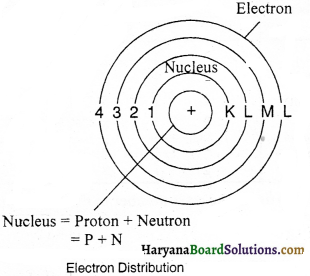This distribution of electrons was conceived by Bohr and Bury. According to them, the valence shell (outermost shell) cannot accommodate more than eight electrons and second last shell cannot accommodate more than 18 electrons.

Example:
Hydrogen has only single orbit where only 1 electron is there. 2 electrons of helium revolve in the first orbit. Lithium has 3 electrons out of which the third electron enters the second shell. Similarly in the atom of calcium there are 20 electrons out of which in the first shell there are 2, in the second and third orbit 8-8 and in the fourth shell there are 2 electrons.Question 11.
Define valency. How is it related to atomic structure?
Valency:
Valency of an atom is the number of valence electrons, whereas valence electrons are the electrons of the valence shell of any atom. The valency of an element and the valency of the electrons present in its outermost shell have the following relationship :
(1) The elements that have 1-4 electrons in their valence shells, their valency is equal to that of the electrons present in the valence shell.

(2) The elements that have 5-8 electrons in their valence shells, their valency is equal to the (8-electrons existing) outermost shell of the element.

(3) In the valence shell of helium, due to two electrons, its outermost shell gets filled up completely, that is why the valency of helium is zero. Similarly, the valency of other active gases is zero because the outermost shells get accommodated.

Normally, valency of metals = number of electrons present in the outermost shell and valency of non-metals = 8- number of electrons present in the outermost shell. Therefore, we can say that valency is related to the atomic structure, since the valency of electrons present in the valence shell is known only by atomic structure.

Question 12.
What are valence electrons? How many valence electrons are there in Mg and Al ?
Valence electrons present in the outermost orbit are known as valence electrons.
The atomic serial number of Mg and A1 is 12 and 13 respectively. Their electronic configuration is:
Mg – 2,8,2
Al – 2,8,3
Therefore, we come to know that the number of valence electrons in Mg and Al is 2 and 3 respectively.

Question 13.
Describe the distribution of electrons in different orbits of the following atoms : lithium, nitrogen, neon, magnesium and silicon.

 Element Atomic number Distribution of Electrons in different shells N K L M Lithium (Li) 3 2 1 Nitrogen (N) 7 2 5 Neon (Ne) 10 2 8 Magnesium (Mg) 12 2 8 2 Silicon (Si) 14 2 8 4

Question 14.
In the given hint 2040Ca , write down the mass number of calcium, atomic number, electronic configuration and atomic structure.
Mass number = 40
Atomic number = 20
Electronic configuration = 2, 8, 8,2

Structure of nucleus :
Number of protons = 20
Number of neutrons = 40 – 20 = 20Question 15.
How many of the following are protons and neutrons ?
14N7 14C6 15P15
(1) 14N7
Atomic mass = 14
Atomic number = 7
Number of protons = 7
Number of neutrons = Atomic mass – atomic number = 14 – 7 = 7

(2) 14C6
Atomic mass = 14
Atomic number = 6
Number of protons = 6
Number of neutrons = Atomic mass – atomic number
= 14 – 6 = 8

(3) 15P15
Atomic mass = 31
Atomic number = 15
Number of protons = 15
Number of neutrons = Atomic mass – atomic number
= 31 – 15 = 16

Question 16.
Explain the chemical neutrality and chemical reactivity of atom.
The chemical properties of an atom depend upon the schematic system of setting up of electrons in it. When the valence shell of an atom of an element gets filled up completely, then that element does not participate in chemical reaction, that is, such an atom is chemically neutral, for example neon, argon, etc. When the outermost shell of an atom of any element is not completely filled up, then such an atom is chemically reactive. The law of chemical reactivity depends upon the distribution system of electrons.

Question 17.
Why are inert or inactive gases behaviourally inactive?
The valence shell in inert gases remains filled up completely. In helium, being the first orbit it gets completed due to two electrons. In rest of the inert gases it gets filled up with 8 electrons. Thereby, the electronic configuration of inert gases used to be stable. The cause of neutrality of these elements is the difficulty of entrance or exit of the electrons. It needs more energy to out electrons out of them more on so they also do not have the efficiency of acquiring electrons.

Question 18.
Boron has two isotopes 5B10 (19%) and 5B11 (81%). Find average atomic weight for Boron.
Average atomic mass of Boron atom = [10 x $$\frac {19}{100}$$ + 11 x $$\frac {81}{100}$$ ]
= $$\left[\frac{190}{100}+\frac{891}{100}\right]$$ = [1.90 + 8.91] = 10.81 = 11

Essay Type Questions

Question 1.
Who discovered the nucleus of atom and how ?
The nucleus of atom was discovered by Ernest Rutherford by experimenting with the scattering of alpha particles.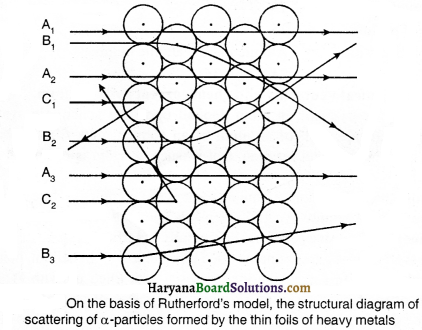In this experiment, Rutherford happened to bombard a high speed stream of alpha particles on a thin foil of gold and he found that after striking the gold leaf, the alpha particles generally deflect from their path by an angle of 1°. One of the smallest parts of the alpha particles get scattered by a larger angular deflecting and come back after getting deflected very little.

It is a general fact that alpha particles are too much energetic particles. Their deflection of forming of bigger angle shows that they had hit against the total mass of atom of the substance. On the basis of this demonstration, he concluded that:

(i) The central part of the atom i. e., the nucleus is solid and has positive charge. The nucleus possesses approximately the total mass of the atom.
(ii) This central part of atom has a very’ small size as compared to the atom itself.Question 2.
What new concept was introduced in Neils Bohr model of an atom by him ?
Neils Bohr proposed a model of atom in 1912 A.D., which was basically based upon the new concept. These concepts are: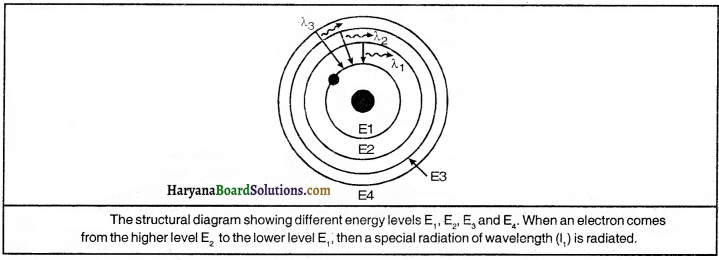(1) Electrons revolve around the nucleus in fixed orbits and each orbit has a different radius.
(2) Each orbit does have its definite energy. Accordingly, the orbit located near the nucleus possesses the least energy and the orbit situated farthest from the nucleus possesses the maximum energy.
(3) When an electron moves to the orbit of a lower energy level from the higher energy level, then the difference of energy is emitted in the form of electromagnetic radiation or in the form of light.
(4) All around the nucleus to fill up the different orbits or shells the number of electrons does have a definite number.
(5) Electrons can constantly revolve in a stable orbit in the form of electromagnetic waves without radiating energy.
(6) If energy is propagated to the electron with a definite energy level, it can enter the orbit with a higher energy level.

Question 3.
What are the similarities in the electronic configuration of the following atoms?
(i) Lithium, Sodium, Potassium
(ii) Helium, Neon, Argon
(iii) Beryllium, Magnesium and Calcium.
(i) Lithium, Sodium, Potassium:

 Element Atomic Number Electron Distribution K L M N Lithium 3 2 1 Sodium 11 2 8 1 Potassium 19 2 8 8 1

In the above electron distribution, we learn that there is only one or single electron in the valence shell of all the three atoms of the elements i.e. Lithium, Sodium and Potassium due to that reason the valency of all these elements is the same.

(ii) Helium, Neon, and Argon:

 Element Atomic Number Electron Distribution K L M N Helium 2 2 Neon 10 2 8 Argon 18 2 -8 8

From the above electron distribution, it is learned that the valence shells (outermost shells) of the atoms of all the three elements i.e., Helium, Neon and Argon are completely filled up, due to that reason their valency is zero or all these elements are inert or inactive.

(iii) Beryllium, Magnesium and Calcium:

 Element Atomic Number Electron Distribution K L M N Beryllium 4 2 2 Magnesium 12 2 8 2 Calcium 20 2 8 8 2

From the above electron distribution we learn that in the atoms of all three elements, there are two electrons in the valence shell. Because of that reason, all the valency of all these elements is 2.

Question 4.
Explain with suitable examples the experimental uses of radioactive isotopes.
Following are the experimental uses of radioactive isotopes :
(1) It can help in fixing the age of the earth, rocks, hills, meteors, etc.
(2) It can be used to find the thickness of paper, glass, plastic, etc.
(3) It can be used in finding out the cracks and crevices in the pipes.
(4) It helps in knowing the wear and tear in the machines.
(5) Isotopes are used in the treatment of different diseases in the medical field; e.g., CO-60 for curing cancer; isotope of iodine for curing thyroid, isotope of phosphorus for leukemia (blood cancer), etc.
(6) The radiations emitted through the radioactive isotopes are used in the form of high disease resistance in growing crops like wheat, paddy and groundnut (peanut).
(7) Isotopes are used in reforming fertilizers.
(8) Isotope Fe-59 is used to examine anemiaQuestion 5.
Show the electronic distribution of the first 20 elements in the Periodic Table.
The electronic distribution of the first 20 elements in the periodic table is:

 Atom Hint Number of Electrons Arrangement of electrons in orbits Distribution of electrons Valency Hydrogen H 1 1 in the first orbit 1 1 Helium He 2 2 in the first orbit 2 2 Lithium Li 3 2 in the first orbit + 1 in the second orbit 2, 1 1 Berylium Be 4 2 in the first orbit + 2 in the second orbit 2,2 2 Boron B 5 2 in the first orbit + 3 in the second orbit 2, 3 3 Carbon C 6 2 in the first orbit + 4 in the second orbit 2,4 4 Nitrogen N 7 2 in the first orbit + 5 in the second orbit 2,5 3 Oxygen 0 8 2 in the first orbit + 6 in the second orbit 2, 6 2 Fluorine F 9 2 in the first orbit + 7 in the second orbit 2,7 1 Neon Ne 10 2 in the first orbit + 8 in the second orbit 2,8 0 Sodium Na 11 2 in the first orbit + 8 in the second orbit+ 1 in the third orbit 2, 8, 1 1 Magnesium Mg 12 2 in the first orbit + 8 in the second orbit + 2 in the third orbit 2, 8,2 2, 8,3 Aluminium Al 13 2 in the first orbit + 8 in the second orbit + 3 in the third orbit 2, 8,3 2, 8,4 Silicon Si 14 2 in the first orbit + 8 in the second orbit + 4 in the third orbit 2, 8,4 2,8,5 Phosphorus P 15 2 in the first orbit + 8 in the second orbit + 5 in the third orbit 2,8,5 2, 8,6 Sulphur s 16 2 in the first orbit + 8 in the second orbit + 6 in the third orbit 2, 8,6 2, 8,7 Chlorine Cl 17 2 in the first orbit + 8 in the second orbit + 7 in the third orbit 2, 8,7 2, 8, 8 Argon Ar 18 2 in the first orbit + 8 in the second orbit + 8 in the third orbit 2, 8, 8 2, 8, 8, 1 Potassium K 19 2 in the first orbit + 8 in the second orbit+ 8 in the third orbit + 1 in the fourth orbit 2, 8, 8, 1 2, 8, 8, 2 Calcium Ca 20 2 in the first orbit + 8 in the second orbit + 8 in the third orbit + 2 in the fourth orbit 2, 8, 8, 2 2, 8,3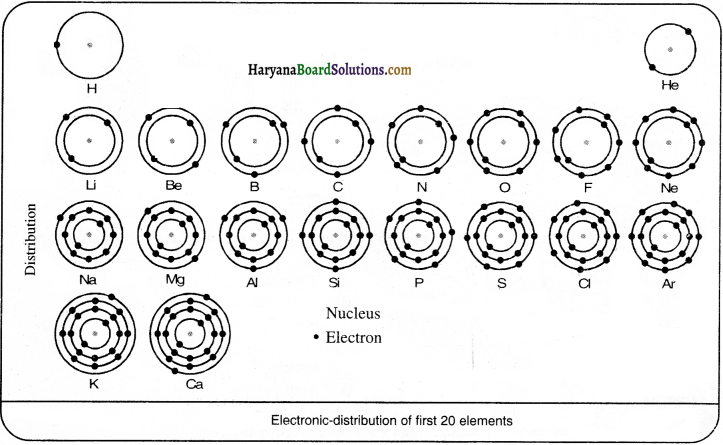Practical Work

Experiment 1:
Prove experimentally that when two bodies are rubbed against each other, charge is produced.

Procedure:
(1) Take a comb and rub it against the dry hair. Bring the comb near to the paper-bits. We notice the comb attracts the paper-bits to it. This proves that on rubbing two bodies against each other, a charge gets produced.

(2) Rub a glass rod with a silken cloth on bringing this glass rod near to the balloon, the balloon will get attracted to the charged glass rod. Thus, the experiment proves that on rubbing the glass rod against the silken cloth, the glass rod gets charged.Experiment 2:
Explain with an experiment Rutherford’s scattering of alpha (a) particles.

Procedure:
To understand Rutherford’s scattering alpha (a ) particles experiment is performed in the open ground. Suppose a child is standing in front of a wall with their eyes shut. Now, ask him to throw some stones against the wall from at a short distance. On striking every stone against the wall, he will hear a sound.

If he repeats the activity ten times, he will hear the sound ten times. But if the child with his eyes shut throws the stones against a wall surrounded with barbed wire, most of the stones will not strike against that boundary and hence, no sound will be heard. As there is enough blank space in between the boundary, through which the stone escapes.

Quick Review of the Chapter

1. Which scientist discovered Canal Rays ?
(A) Dalton
(B) Li. Thomson
(C) E. Goldstein
(D) Neils Bohr
(C) E. Goldstein

2. Which scientist discovered electrons ?
(A) Thomson
(B) Dalton
(C) Goldstein
(D) Neils Bohr
(A) Thomson

3. When was J.J. Thomson awarded Nobel Prize ?
(A) in 1878
(B) in 1902
(C) in 1906
(D) in 1912
(C) in 1906

4. Which scientist proposed that an atom consists of positively charged sphere and the electrons are embedded in it ?
(A) Rutherford
(B) Thomson
(C) Dalton
(D) Neils Bohr
(B) Thomson5. E. Rutherford discovered
(A) atom
(B) nucleus
(C) proton
(D) neutron
(B) nucleus

6. The radius of nucleus is times than the radius of an atom.
(A) 105
(B) 104
(C) 103
(D) 102
(A) 105

7. Which scientist told that when electrons revolve in discrete orbits, then energy ?
(A) Neils Bohr
(B) Rutherford
(C) Dalton
(D) Thomson
(A) Neils Bohr

8. Who is the author of the book ‘Atomic Theory’ ?
(A) Rutherford
(B) Thomson
(C) Neils Bohr
(D) both (A) and (B)
(C) Neils Bohr

9. Charge on nucleus of an atom is:
(A) zero charge
(B) negative charge
(C) positive charge
(D) no charge
(C) positive charge

10. When was neutron discovered?
(A) in 1902
(B) in 1912
(C) in 1922
(D) in 1932
(D) in 1932

11. Who discovered neutron?
(B) J ,J. Thomson
(C) Neil Bohr
(D) None of the above

12. The characteristic of electron is not:
(A) uncharged
(B) mass same as proton
(C) found in nucleus
(D) it has negative charge
(D) it has negative charge

13. The characteristic of electron is not:
(A) positively charged
(B) represents by e-
(C) negatively charged
(D) lightest particle
(A) positively charged14. The characteristic of proton is not:
(A) found in nucleus
(B) represents by p+
(C) mass 1 unit
(D) negatively charged
(D) negatively charged

15. The first electron shell of an atom can have maximum electrons.
(A) 2
(B) 8
(C) 18
(D) 32
(A) 2

16. The maximum number of electrons in third electron shell can be:
(A) 8
(B) 12
(C) 18
(D) 32
(C) 18

17. The number of electrons in fourth electron shell can be:
(A) 8
(B) 18
(C) 32
(D) 2
(C) 32

18. What will be the atomic number of an element having 6 electrons in its M shell?
(A) 8
(B) 16
(C) 24
(D) 34
(B) 16

19. The number of electrons present in outermost shell of neon:
(A) 2
(B) 8
(C) 18
(D) 32
(B) 820. Which of the following element is inert gas?
(A) neoft
(B) argon
(C) cripton
(D) all of the above
(D) all of the above

21. The number of electrons present in the outermost shell of fluorine
(A) 2
(B) 5
(C) 7
(D) 17
(C) 7

22. The number of electrons in electrons in outermost shell can be:
(A) 2
(B) 8
(C) 18
(D) 32
(B) 8

23. What is the number of neutrons in hydrogen
(A) zero
(B) one
(C) two
(D) four
(A) zero

24. Protium is an isotope of which element ?
(A) helium
(B) hydrogen
(C) lithium
(D) nitrogen
(B) hydrogen

25. The symbol of tritium is:
(A) 11H
(B) 12H
(C) 13H
(D) 14H
(C) 13H26. Deuterium is an isotope of which element?
(A) uranium
(B) lithium
(C) helium
(D) hydrogen
(D) hydrogen

27. …………. is used as fuel in atomic furnace.
(A) Lithium
(B) Helium
(C) Uranium
(D) Beryllium
(C) Uranium

28. The isotope of which element is used in the treatment of cancer ?
(A) Cobalt
(B) Iodine
(C) Boron
(D) Chlorine
(A) Cobalt

29. the isotope of iodine is used in the treatment of ……………. disease
(A) atomic nucleus
(B) electron
(C) proton
(D) neutron
(A) atomic nucleus

30. If K and L shell of an atom are full, then the number of electrons in it will be:
(A) 16
(B) 8
(C) 17
(D) 18
(D) 18

31. For Which invection is Rutherfords alpha scattering experement responsible?
(A) atomic nucleus
(B) electron
(C) proton
(D) neutron
(A) atomic nucleus32. The number of electrons in Cl ion is:
(A) 16
(B) 8
(C) 17
(D) 18
(D) 18

33. Which is correct electronic configuration of sodium ?
(A) 2, 8
(B) 8, 2, 1
(C) 2,8,1
(D) 8,2
(C) 2, 8, 1

34. The fundamental particle of an atom is not:
(A) electron
(B) positron
(C) proton
(D) neutron